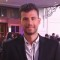Home » Core Java » class » Static inner class exampleIlias is a software developer turned online entrepreneur. He is co-founder and Executive Editor at Java Code Geeks.

# Static inner class example

This is an example of how to use a static inner class. We have set the example as described below:

• We have created a class, `ArAlgo` that contains a static inner class `P`.
• Class `P` has two double attributes and their getters.
• `ArAlgo` class also has a static method,` P min_max(double[] vals)`. The method gets a double array and for each value in the array and computes the minimum
and the maximum value in the array.
• We create a new double array and fill it with random values, using `random()` API method of Math. Then we get a new instance of `P` class, using `min_max(double[] vals)` method of `ArAlgo` class, with the double array created above. We use the getters of the `ArAlgo` class to get the values of the two fields.

Let’s take a look at the code snippet that follows:

```package com.javacodegeeks.snippets.core;

public class StaticInnerClass {

public static void main(String[] args) {

double[] num = new double;

for (int i = 0; i < num.length; i++) {

num[i] = 100 * Math.random();

}

ArAlgo.P p = ArAlgo.min_max(num);

System.out.println("min = " + p.getF());

System.out.println("max = " + p.getS());
}
}

class ArAlgo {

/**
* A pair of floating-point numbers
*/
public static class P {

/**

* Constructs a pair from two floating-point numbers

*/

private double f;

private double s;

public P(double a, double b) {

f = a;

s = b;

}

/**

* Returns the first number of the pair

*/

public double getF() {

return f;

}

/**

* Returns the second number of the pair

*/

public double getS() {

return s;

}
}

/**
* Computes both the minimum and the maximum of an array
*/
public static P min_max(double[] vals) {

double min = Double.MAX_VALUE;

double max = Double.MIN_VALUE;

for (double v : vals) {

if (min > v) {

min = v;

}

if (max < v) {

max = v;

}

}

return new P(min, max);
}
}
```

Output:

``````min = 1.5117631236976625
max = 90.86550459529965``````

This was an example of static inner class in Java.

# Do you want to know how to develop your skillset to become a Java Rockstar?

## Subscribe to our newsletter to start Rocking right now!

### and many more ....